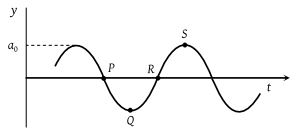NEET Physics Waves Questions Solved

A wave motion has the function $y={a}_{0}\mathrm{sin}\left(\omega \text{\hspace{0.17em}}t-kx\right)$. The graph in figure shows how the displacement y at a fixed point varies with time t. Which one of the labelled points shows a displacement equal to that at the position $x=\frac{\pi }{2k}$ at time t = 0(1) P

(2) Q

(3) R

(4) S

Explanation is a part of a Paid Course. To view Explanation Please buy the course.

Difficulty Level: